# Electrical and Control Engineering: Transfer Function Reduction problem

• Engineering
New poster has been reminded to show their work on schoolwork problems
Homework Statement:
I am having a problem with calculating transfer function given with a picture below i need to calculate output Y(p) / input V(p) analitically but i dont know how to do it
Thanks for any help or comment
Relevant Equations:
Transfer function reduction analiticall equations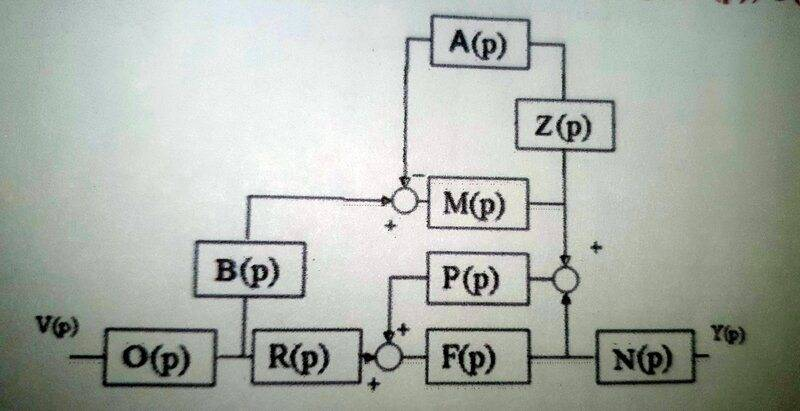FactChecker
Gold Member
You should show some work and then we can give hints. Replace feedback loops with single blocks. To do that, you might need to split off some feedback loops (F,P) from feed-forward paths (B,M,P). Replacing the feedback loops will leave you with only feed-forward paths which should be easier to deal with.

Last edited:
•berkeman
You should show some work and then we can give hints. Replace feedback loops with single blocks. To do that, you might need to split off some feedback loops (F,P) from feed-forward paths (B,M,P). Replacing the feedback loops will leave you with only feed-forward paths which should be easier to deal with.
I know how to solve this problem grafically, but this problem i need to solve analitically, without reducing loops.

DaveE
Gold Member
I know how to solve this problem grafically, but this problem i need to solve analitically, without reducing loops.
I'm not sure exactly what "analytical" means in this context. However, you could do it using brute force. Give a name to every node and just write out all of the equations relating them. You'll have a bunch of "analytical" equations, that you can reduce to a solution.

•berkeman
Joshy
Gold Member
Want to try Mason’s gain? Try a few things out like listing then forward path and each loop.

FactChecker
Gold Member
I know how to solve this problem grafically, but this problem i need to solve analitically, without reducing loops.
I don't know how you are distinguishing an "analytical" solution. IMO, because the blocks are only identified symbolically, any solution is analytical. If you show some work or reference to an example it might be clear what you mean.

I don't know how you are distinguishing an "analytical" solution. IMO, because the blocks are only identified symbolically, any solution is analytical. If you show some work or reference to an example it might be clear what you mean.
Here is the example solved analitically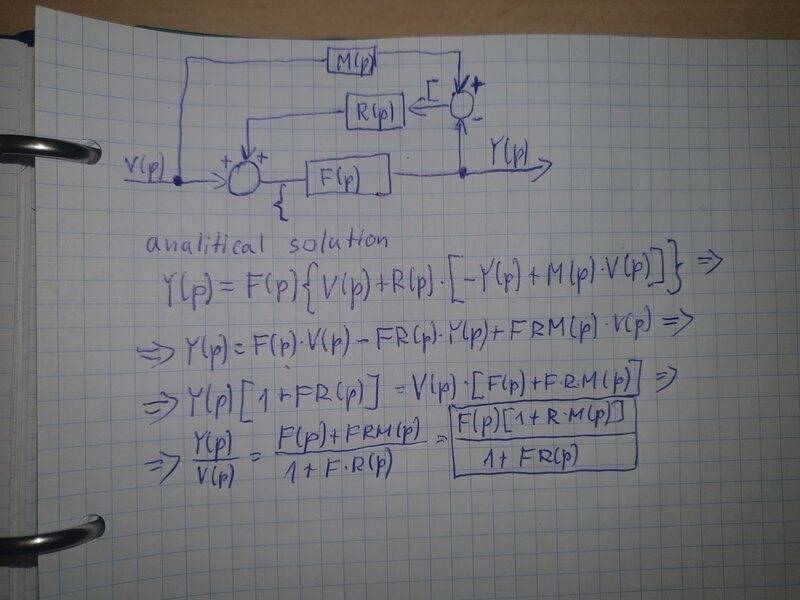FactChecker
Gold Member
Ok. What you are calling graphical manipulations are mirrored by analytical, symbolic manipulations. Reducing a feedback loop to a single block is expressed analytically. The single block replacement of the feedback loop has an analytical expression in it. The process of the graphical solution can give you the steps to get the desired analytical expression.

•AncientOne99
DaveE
Gold Member
Here is the example solved analiticallyView attachment 291213
This example is only a little less complicated than your original problem. Is this your work or a copy of a worked example? I'm a little confused about what your problem is, please explain what confuses you and show an attempted solution. We can't help if we don't know what part is confusing you.

Below I've shown an analytical solution to the trickiest part of these networks. Notice that I've given arbitrary names to the internal nodes that weren't already named. That allows us to construct one equation for each block. In the example above, you will have 5 equations. After you have those equations you eliminate all of those internal variables by substitution.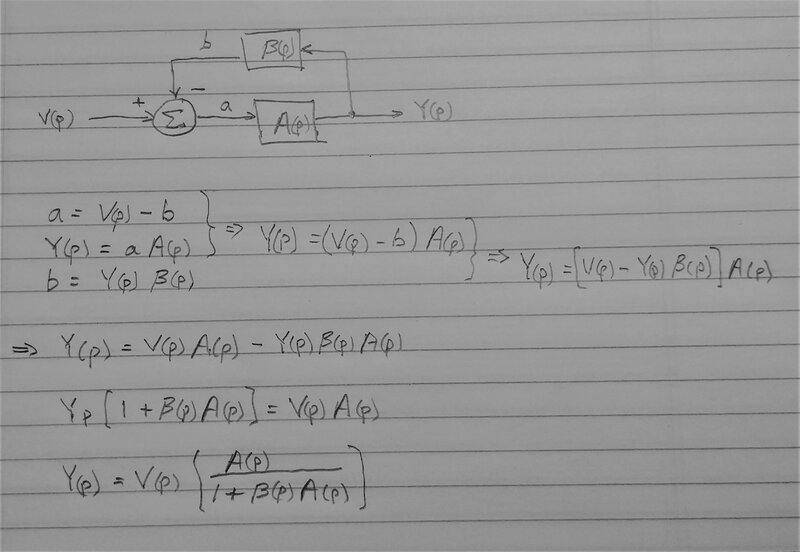•FactChecker
Here is my attempt for trying to solve TF analitically, i dokt know what to do after that second sumator.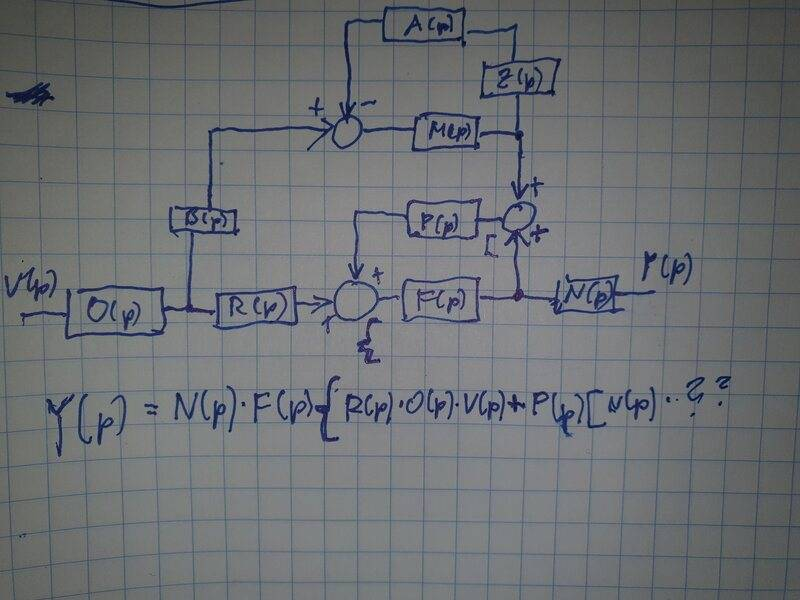FactChecker
Gold Member
These can be very hard to write down in one step. That is why I would recommend an analytical approach of a few simpler steps.
1) Replace the M->Z->A feedback loop with a single block, M' = M/(1+AMZ)
2) Duplicate the P block so that there are two separate paths: a forward path M'->P->F and a feedback loop, P->F->P. Then replace the second loop with a single block, F' = F/(1-PF).
3) Now you have only forward paths with no loops and can just sum and multiply them at the appropriate places.
@DaveE 's post #9 shows a more methodical approach that would help greatly in complicated examples.

These can be very hard to write down in one step. That is why I would recommend an analytical approach of a few simpler steps.
1) Replace the M->Z->A feedback loop with a single block, M' = M/(1+AMZ)
2) Duplicate the P block so that there are two separate paths: a forward path M'->P->F and a feedback loop, P->F->P. Then replace the second loop with a single block, F' = F/(1-PF).
3) Now you have only forward paths with no loops and can just sum and multiply them at the appropriate places.
@DaveE 's post #9 shows a more methodical approach that would help greatly in complicated examples.
I have solved this problem, but i dont know if it is correct, can you please tell me if i am right. Thanks for support.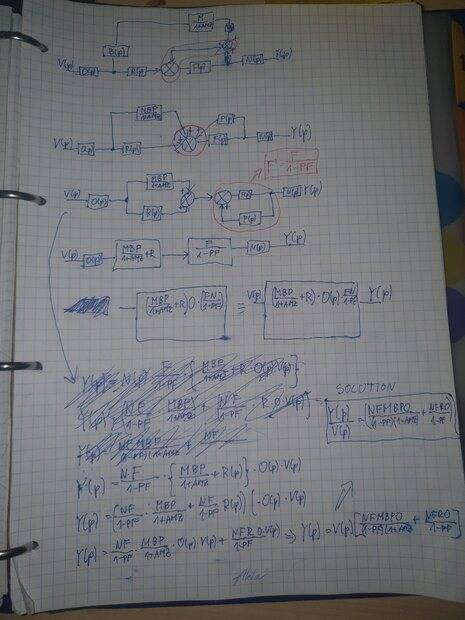FactChecker
Gold Member
I have trouble reading the details, but I think that I agree with it.

I'm thinking about steps for solving this step analytically but i dont really know the othher equasions in order to calculate output/input here are the equasions that i was given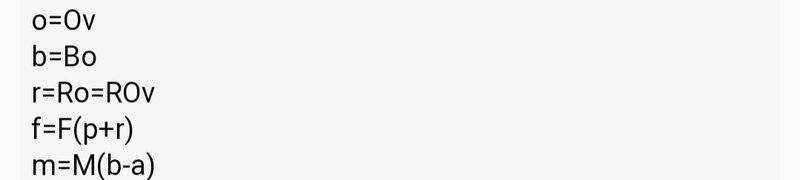FactChecker
Gold Member
What about p=P(z+m+f)? This must be done methodically. Label each signal on a diagram and make sure that you have an equation for each one. Do not try to keep track of too much in your head, it will frequently lead to mistakes.

I don't understand methodical way, can you please correct me if i am wrong. Thank you.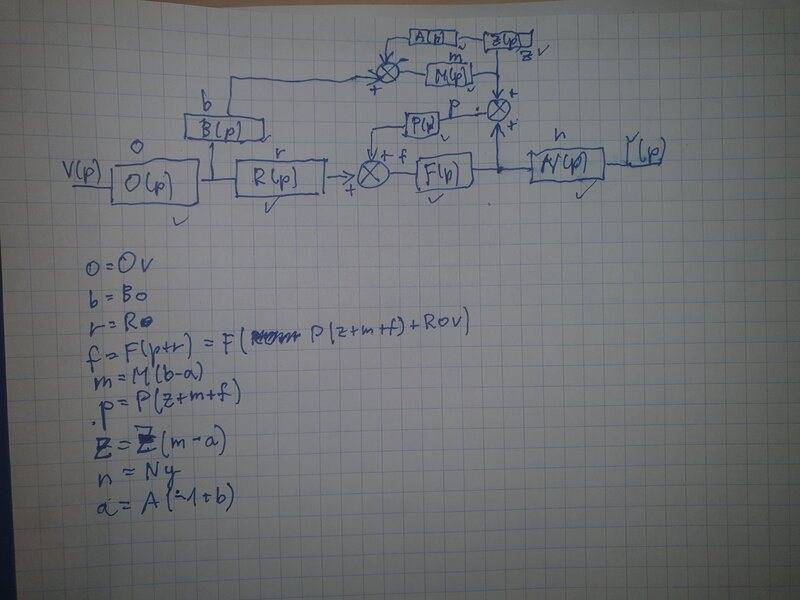FactChecker
Gold Member
You need to use a methodical labeling method. I assumed that you were always labeling the output signal of a block. On the F->P feedback loop, I think you labeled the input signal rather than the output signal of P. The 'p' label is on the wrong side of the P block. Also, I don't think I agree with your equation for the 'a' signal.

This approach gives you a lot of simultaneous linear equations. I think that some linear matrix methods can be applied to simplify the equations.

You need to use a methodical labeling method. I assumed that you were always labeling the output signal of a block. On the F->P feedback loop, I think you labeled the input signal rather than the output side of P. The 'p' label is on the wrong side of the P block. Also, I don't agree with your equation for the 'a' signal.

This approach gives you a lot of simultaneous linear equations. I think that some linear matrix methods can be applied to simplify the equations.
How do i write equasion for output then, even if i wrote for a correctly, i cannot resolve for output signal n = N * y, its only one equasion

FactChecker
Gold Member
This approach will only work if you are very systematic about your labels. You don't need an 'n' for the output of the N block because that is Y. And 'f' is the output of the F block, not the input. Then you start with Y= fN and start replacing things: Y=(F(p+r))N. Now I see that there are confusing labels and equations. I think you need to check all the labels and equations before you can proceed.

But i need to write Y(output)/V(input) function, these equasions are very confusing.
Y= fN = (F(p+r))N = F(P(z+m+f)+ROv)
p=P(z+m+f)
r = Ro=ROv
b = Bo=BOv
o = Ov
f = F(p+r)
m = M(b-a)
a = A ???
z = Z ???

Can you please show me the solution for a and z, thank you, this methodical way is quite hard.
Thanks for support

FactChecker
Gold Member
I think you can not proceed until you check a few things:
1) Do all your signal labels like 'p' refer to the OUTPUT signal of the P block? I think you have a mixture, which is bad.
2) After completing (1), check all your equations.

Why do i need to refer all signals to the output, if i refer them to the output then i wont have any equasions for the input and i wont be able to calculate Y/V

FactChecker
Gold Member
The only difference between the equation of the input and the output signals of block 'X' is the X multiplier. If you have a mixture of labeling inputs and outputs, then you run the risk of missing one of the block multipliers from your equations. I tried to give you a hint of how to work with your equations but I think I ran into a missing block multiplier.

DaveE
Gold Member
Why do i need to refer all signals to the output, if i refer them to the output then i wont have any equasions for the input and i wont be able to calculate Y/V
Look at the simpler example in post #9 above. There are 3 blocks/summers and thus three equations. Also, there are 4 nodes which are all of the variables in those equations. So, 3 equations and 4 unknowns. When you reduce this the way you learned in your algebra classes, you will be left with any one variable (of your choice) expressed in terms of any other variable (of your choice). So you will choose to eliminate all variables except the output and the input.

Look carefully at that example and make sure you completely understand it before you try this on larger networks. The concept is the same for all of these problems, and is mostly an exercise in being careful and methodical in naming things, identifying the equations that relate them, and then solving the system of multiple linear equations. Bigger networks just have more equations and variables. That is the analytical approach. People often make some shortcuts AFTER they are familiar with the process, but I'm not sure you should skip any steps at this point. I suspect that is the source of much of your confusion.

•FactChecker
FactChecker